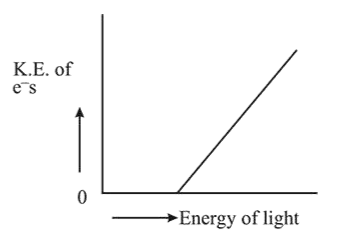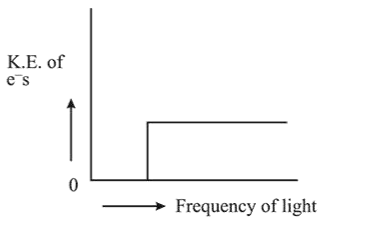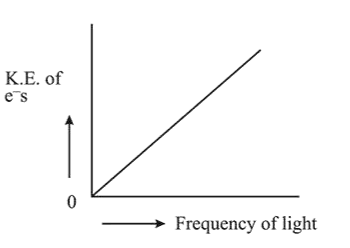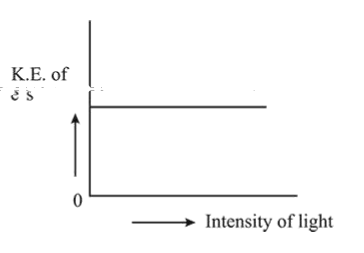# Which of the graphs shown below does not represent theQuestion:

Which of the graphs shown below does not represent the relationship between incident light and the electron ejected from metal surface?

1.2.3.4.Correct Option: , 3

Solution:

K.E. $=\mathrm{h} v-\mathrm{hv}_{0}$ where, $v=$ Frequency of incident radiation $v_{0}=$ Threshold frequency

KE is independent of intensity, it depends on frequency of light. Intensity is directly proportional to the no, of electrons emitted.